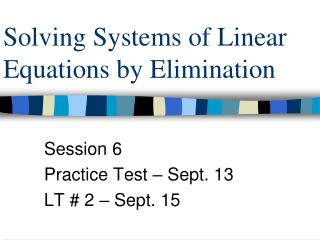DownloadDownload PresentationSolving Systems of Linear Equations by Elimination

# Solving Systems of Linear Equations by Elimination

Download Presentation## Solving Systems of Linear Equations by Elimination

- - - - - - - - - - - - - - - - - - - - - - - - - - - E N D - - - - - - - - - - - - - - - - - - - - - - - - - - -
##### Presentation Transcript

1. Solving Systems of Linear Equations by Elimination Session 6 Practice Test – Sept. 13 LT # 2 – Sept. 15

2. Objective • Solve a system of linear equations in two variables by the elimination method.

3. 3x – y = 12 (1) 2x + y = 13 (2) + 5x = 25 x = 5 By what operation can we eliminate a variable in the given system?

4. 3x – y = 12 (1) 2x + y = 13 (2) + 5x = 25 x = 5 (5, 3) 3(5) – y = 12 y = 3 How do we find the value of y?

5. 3x – y = 12 (1) 2x + y = 13 (2) + 5x = 25 x = 5 (5, 3) 3(5) – y = 12 y = 3 How do we know if the values of x and y are correct?

6. 3x – y = 12 (1) 2x + y = 13 (2) (5, 3) 2(5) + 3 = 13 3(5) – 3 = 12 15 – 3 = 12 10 + 3 = 13 13 = 13 12 = 12 (5, 3) How do we know if the values of x and y are correct?

7. Example 1 page 154 3x + 7y = 17 3x - 6y = 4

8. Example 2 page 155 4x -5y = 17 x - 5y =8

9. SYSTEMS THAT HAVE ONLY ONE SOLUTION (Consistent, Independent) x – 2y = -6 4x + 3y = 20

10. SYSTEMS THAT HAVE NO SOLUTION (Inconsistent) y = -1/2x + 2 y = -1/2x + 3

11. SYSTEMS THAT HAVE NO SOLUTION (Inconsistent) 2x – 3y = 6 6x – 9y = 36

12. SYSTEMS THAT HAVE MORE THAN ONE SOLUTION (Consistent, Dependent) y = -3x + 1 y = -3x + 1

13. SYSTEMS THAT HAVE MORE THAN ONE SOLUTION (Consistent, Dependent) 4x + 6y = 4 6x + 9y = 6